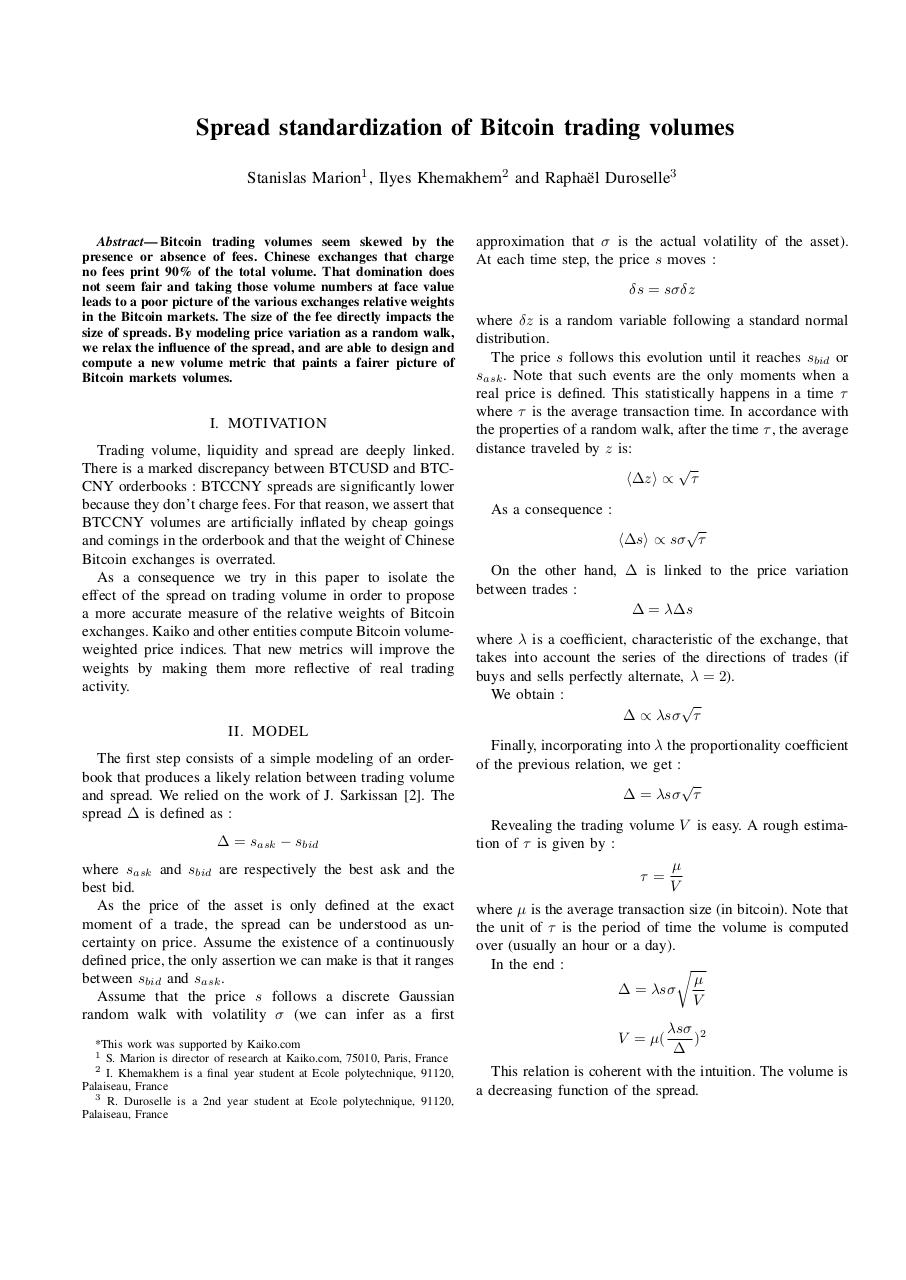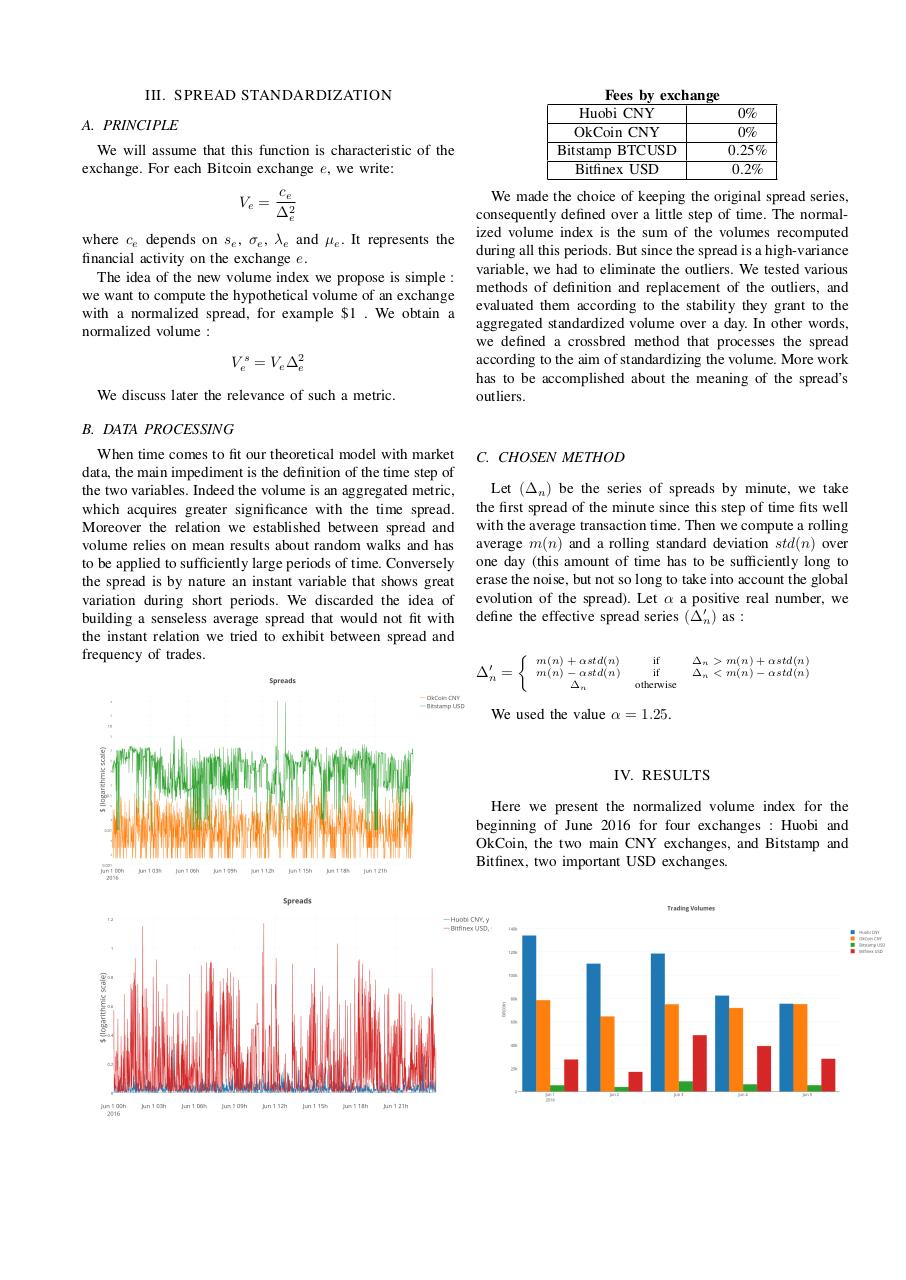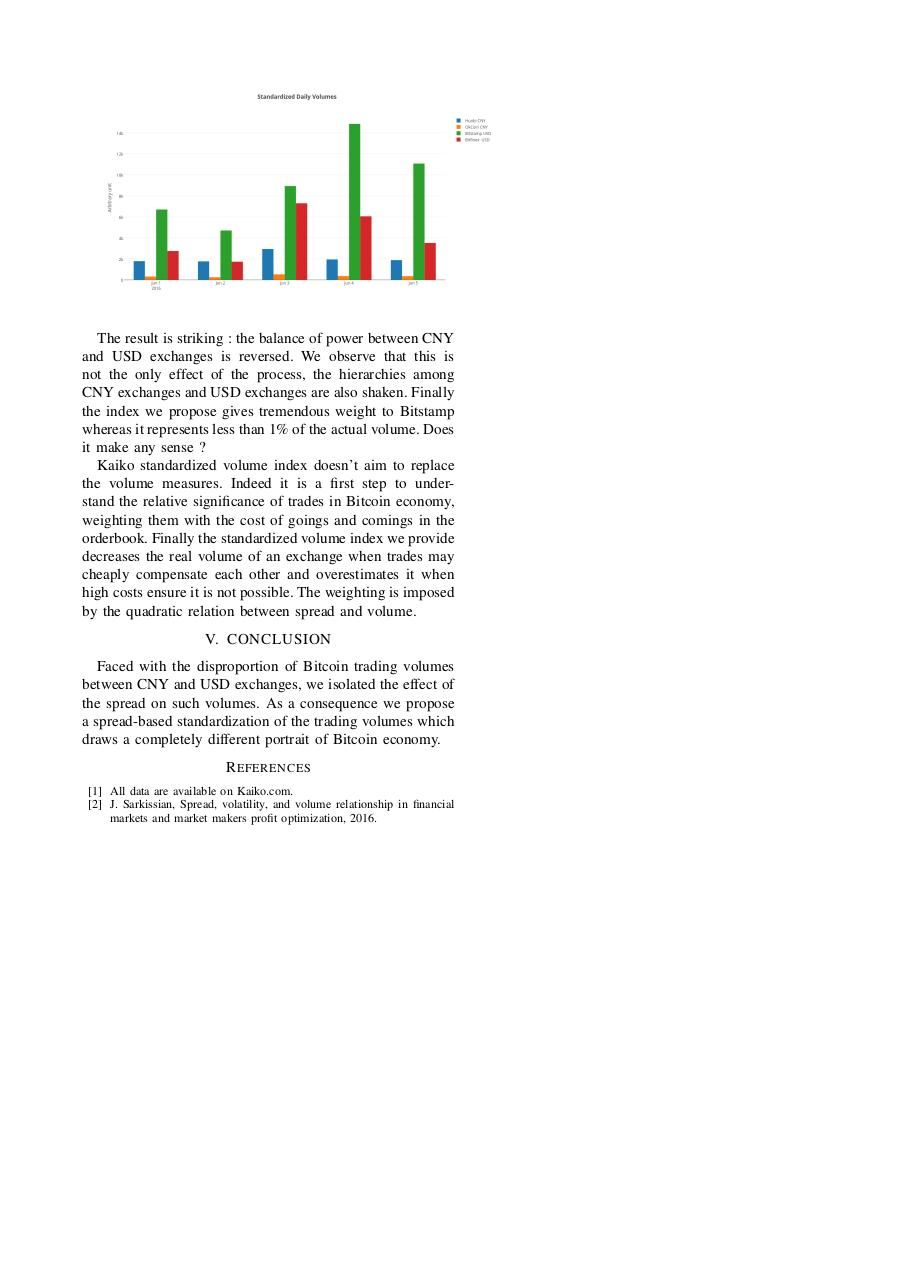### File information

This PDF 1.5 document has been generated by TeX / pdfTeX-1.40.16, and has been sent on pdf-archive.com on 21/01/2017 at 15:38, from IP address 82.234.x.x. The current document download page has been viewed 275 times.
File size: 454 KB (3 pages).
Privacy: public file

### Document preview

Stanislas Marion1 , Ilyes Khemakhem2 and Rapha¨el Duroselle3

Abstract— Bitcoin trading volumes seem skewed by the
presence or absence of fees. Chinese exchanges that charge
no fees print 90% of the total volume. That domination does
not seem fair and taking those volume numbers at face value
leads to a poor picture of the various exchanges relative weights
in the Bitcoin markets. The size of the fee directly impacts the
size of spreads. By modeling price variation as a random walk,
we relax the influence of the spread, and are able to design and
compute a new volume metric that paints a fairer picture of
Bitcoin markets volumes.

I. MOTIVATION
There is a marked discrepancy between BTCUSD and BTCCNY orderbooks : BTCCNY spreads are significantly lower
because they don’t charge fees. For that reason, we assert that
BTCCNY volumes are artificially inflated by cheap goings
and comings in the orderbook and that the weight of Chinese
Bitcoin exchanges is overrated.
As a consequence we try in this paper to isolate the
a more accurate measure of the relative weights of Bitcoin
exchanges. Kaiko and other entities compute Bitcoin volumeweighted price indices. That new metrics will improve the
weights by making them more reflective of real trading
activity.

approximation that σ is the actual volatility of the asset).
At each time step, the price s moves :
δs = sσδz
where δz is a random variable following a standard normal
distribution.
The price s follows this evolution until it reaches sbid or
sask . Note that such events are the only moments when a
real price is defined. This statistically happens in a time τ
where τ is the average transaction time. In accordance with
the properties of a random walk, after the time τ , the average
distance traveled by z is:

h∆zi ∝ τ
As a consequence :

h∆si ∝ sσ τ
On the other hand, ∆ is linked to the price variation
∆ = λ∆s
where λ is a coefficient, characteristic of the exchange, that
takes into account the series of the directions of trades (if
buys and sells perfectly alternate, λ = 2).
We obtain :

∆ ∝ λsσ τ

II. MODEL
The first step consists of a simple modeling of an orderbook that produces a likely relation between trading volume
and spread. We relied on the work of J. Sarkissan . The
spread ∆ is defined as :
best bid.
As the price of the asset is only defined at the exact
moment of a trade, the spread can be understood as uncertainty on price. Assume the existence of a continuously
defined price, the only assertion we can make is that it ranges
Assume that the price s follows a discrete Gaussian
random walk with volatility σ (we can infer as a first
*This work was supported by Kaiko.com
1 S. Marion is director of research at Kaiko.com, 75010, Paris, France
2 I. Khemakhem is a final year student at Ecole polytechnique, 91120,
Palaiseau, France
3 R. Duroselle is a 2nd year student at Ecole polytechnique, 91120,
Palaiseau, France

Finally, incorporating into λ the proportionality coefficient
of the previous relation, we get :

∆ = λsσ τ
Revealing the trading volume V is easy. A rough estimation of τ is given by :
µ
τ=
V
where µ is the average transaction size (in bitcoin). Note that
the unit of τ is the period of time the volume is computed
over (usually an hour or a day).
In the end :
r
µ
∆ = λsσ
V
V = µ(

λsσ 2
)

This relation is coherent with the intuition. The volume is
a decreasing function of the spread.

Fees by exchange
Huobi CNY
0%
OkCoin CNY
0%
Bitstamp BTCUSD
0.25%
Bitfinex USD
0.2%

A. PRINCIPLE
We will assume that this function is characteristic of the
exchange. For each Bitcoin exchange e, we write:
Ve =

ce
∆2e

where ce depends on se , σe , λe and µe . It represents the
financial activity on the exchange e.
The idea of the new volume index we propose is simple :
we want to compute the hypothetical volume of an exchange
with a normalized spread, for example \$1 . We obtain a
normalized volume :
Ves = Ve ∆2e
We discuss later the relevance of such a metric.

consequently defined over a little step of time. The normalized volume index is the sum of the volumes recomputed
during all this periods. But since the spread is a high-variance
variable, we had to eliminate the outliers. We tested various
methods of definition and replacement of the outliers, and
evaluated them according to the stability they grant to the
aggregated standardized volume over a day. In other words,
we defined a crossbred method that processes the spread
according to the aim of standardizing the volume. More work
outliers.

B. DATA PROCESSING
When time comes to fit our theoretical model with market
data, the main impediment is the definition of the time step of
the two variables. Indeed the volume is an aggregated metric,
which acquires greater significance with the time spread.
Moreover the relation we established between spread and
volume relies on mean results about random walks and has
to be applied to sufficiently large periods of time. Conversely
the spread is by nature an instant variable that shows great
variation during short periods. We discarded the idea of
building a senseless average spread that would not fit with
the instant relation we tried to exhibit between spread and

C. CHOSEN METHOD
Let (∆n ) be the series of spreads by minute, we take
the first spread of the minute since this step of time fits well
with the average transaction time. Then we compute a rolling
average m(n) and a rolling standard deviation std(n) over
one day (this amount of time has to be sufficiently long to
erase the noise, but not so long to take into account the global
evolution of the spread). Let α a positive real number, we
define the effective spread series (∆0n ) as :
∆0n


=

m(n) + αstd(n)
m(n) − αstd(n)
∆n

if
if
otherwise

∆n &gt; m(n) + αstd(n)
∆n &lt; m(n) − αstd(n)

We used the value α = 1.25.

IV. RESULTS
Here we present the normalized volume index for the
beginning of June 2016 for four exchanges : Huobi and
OkCoin, the two main CNY exchanges, and Bitstamp and
Bitfinex, two important USD exchanges.

The result is striking : the balance of power between CNY
and USD exchanges is reversed. We observe that this is
not the only effect of the process, the hierarchies among
CNY exchanges and USD exchanges are also shaken. Finally
the index we propose gives tremendous weight to Bitstamp
whereas it represents less than 1% of the actual volume. Does
it make any sense ?
Kaiko standardized volume index doesn’t aim to replace
the volume measures. Indeed it is a first step to understand the relative significance of trades in Bitcoin economy,
weighting them with the cost of goings and comings in the
orderbook. Finally the standardized volume index we provide
decreases the real volume of an exchange when trades may
cheaply compensate each other and overestimates it when
high costs ensure it is not possible. The weighting is imposed
V. CONCLUSION
Faced with the disproportion of Bitcoin trading volumes
between CNY and USD exchanges, we isolated the effect of
the spread on such volumes. As a consequence we propose
draws a completely different portrait of Bitcoin economy.
R EFERENCES
 All data are available on Kaiko.com.
 J. Sarkissian, Spread, volatility, and volume relationship in financial
markets and market makers profit optimization, 2016.#### HTML Code

Copy the following HTML code to share your document on a Website or Blog

#### QR Code### Related keywords